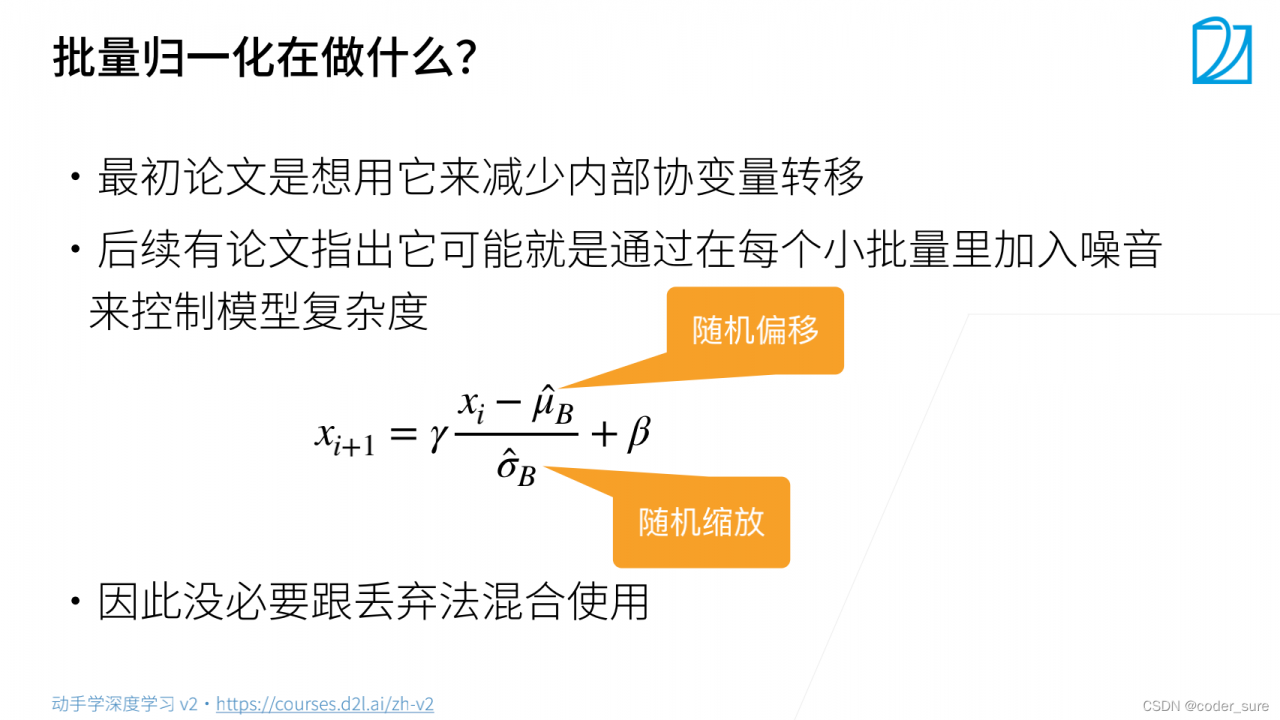# 一、批量归一化

• 损失出现在最后，后面的层训练较快
• 数据在最底端
• 底部的层训练较慢
• 底部层一变化，所有都跟着变，顶部的就相当于白训练啦
• 最后的那些层需要重新学习多次
• 导致收敛变慢
• 我们可以在学习底部层的时候避免变化顶部嘛？

# 二、批量归一化的核心思想

• 固定小批量里面的均值和方差
μ ^ B = 1 ∣ B ∣ ∑ x ∈ B x , σ ^ B 2 = 1 ∣ B ∣ ∑ x ∈ B ( x − μ ^ B ) 2 + ϵ . \begin{aligned} \hat{\boldsymbol{\mu}}_\mathcal{B} &= \frac{1}{|\mathcal{B}|} \sum_{\mathbf{x} \in \mathcal{B}} \mathbf{x},\\ \hat{\boldsymbol{\sigma}}_\mathcal{B}^2 &= \frac{1}{|\mathcal{B}|} \sum_{\mathbf{x} \in \mathcal{B}} (\mathbf{x} - \hat{\boldsymbol{\mu}}_{\mathcal{B}})^2 + \epsilon.\end{aligned}
然后再做额外的调整（可学习的参数）
B N ( x ) = γ ⊙ x − μ ^ B σ ^ B + β . \mathrm{BN}(\mathbf{x}) = \boldsymbol{\gamma} \odot \frac{\mathbf{x} - \hat{\boldsymbol{\mu}}_\mathcal{B}}{\hat{\boldsymbol{\sigma}}_\mathcal{B}} + \boldsymbol{\beta}.

# 三、批量归一化

• 可学习的参数为γ \gammaB \mathcal{B}
• 作用在
• 全连接层和卷积层输出上，激活函数前
• 全连接层和卷积层输入上
• 对全连接层，作用在特征维
• 对于卷积层，作用在通道维

# 四、批量归一化在做什么？# 五、总结

• 批量归一化固定小批量中的均值和方差，然后学习出合适的偏移和缩放
• 可以加速收敛速度，但一般不改变模型精度